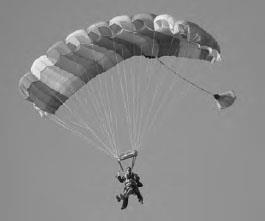NextPrevious

# On what does the terminal velocity depend?

Air drag depends on the density of the air, the size, velocity, and the shape of the object. Therefore the terminal velocity will depend on all of these, plus the mass. You can test to see if the air drag depends on the velocity or the velocity squared by doing a simple experiment. You’ll need only five filters for a drip coffee maker. The filters must be cup-shaped. If the drag depends on velocity, then the terminal velocity will be given by kv = mg, where k is some constant. Thus v will be proportional to m. On the other hand, if the drag depends on the square of the velocity then the terminal velocity will be given by kv2 = mg, so v will be proportional to the square root of the mass.

The experiment depends on the fact that you can compare two velocities by dropping two objects at the same time, allowing them to fall different distances, and observing whether they hit the ground at the same time. If the drag depends on velocity, then doubling the mass will double the velocity. Stack two filters and hold them above your head. Hold a single filter in the other hand half that distance above the ground. Drop them at the same time and see if they hit the ground together, as they should if two filters fall twice as far in the same time. What did you observe? You probably found that they didn’t hit the ground at the same time. Now add two more filters so you have a total of four. The square root of four is two, so if the drag depends on the square of the velocity, the four filters will now fall twice as fast as the single filter. Try it. Do they hit the ground at the same time?

Air drag is more complicated than this simple experiment suggests. At slow speeds the drag constant, k, is larger than at higher speeds. Many studies have been done on the air drag of tennis balls, baseballs, and soccer balls. The fuzz, stitches, and panels have a strong effect on air drag, as anyone who has tried to hit a knuckle ball will testify.The force of the air on this person’s parachute—the air drag—increases as speed increases, and eventually the force of gravity and the upward force of the air equalize, resulting in a constant speed called the terminal velocity.
Close

This is a web preview of the "The Handy Physics Answer Book" app. Many features only work on your mobile device. If you like what you see, we hope you will consider buying. Get the App